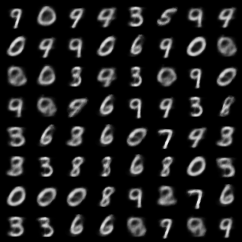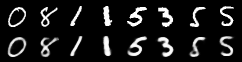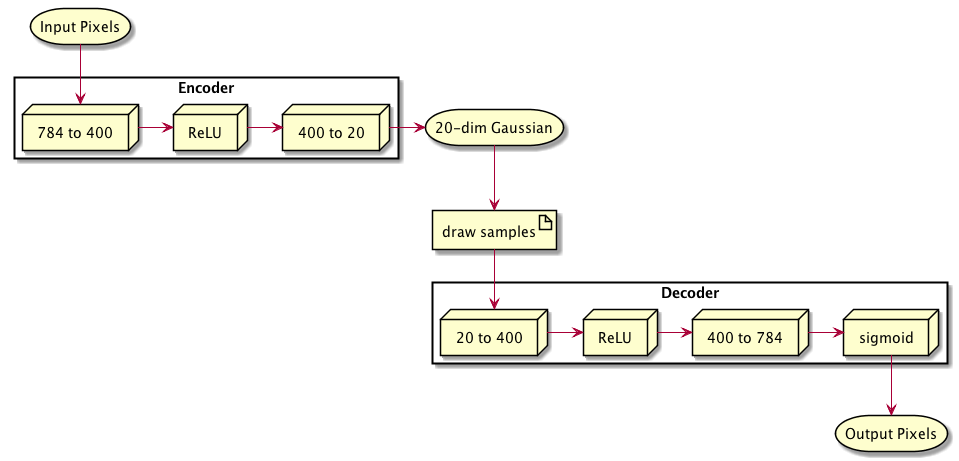I have recently become fascinated with (Variational) Autoencoders and with PyTorch.

Kevin Frans has a beautiful blog post online explaining variational autoencoders, with examples in TensorFlow and, importantly, with cat pictures. Jaan Altosaar’s blog post takes an even deeper look at VAEs from both the deep learning perspective and the perspective of graphical models. Both of these posts, as well as Diederik Kingma’s original 2014 paper Auto-Encoding Variational Bayes, are more than worth your time.

In my case, I wanted to understand VAEs from the perspective of a PyTorch implementation. I started with the VAE example on the PyTorch github, adding explanatory comments and Python type annotations as I was working my way through it.

This post summarises my understanding, and contains my commented and annotated version of the PyTorch VAE example. I hope it helps!

# What is PyTorch?

PyTorch is FAIR’s (that’s Facebook AI Research) Python dynamic deep learning / neural network library. The way that FAIR has managed to make neural network experimentation so dynamic and so natural is nothing short of miraculous. Read this post by fast.ai to find out more about their reasons for excitement, many of which I share.

# What is an autoencoder?

The general idea of the autoencoder (AE) is to squeeze information through a narrow bottleneck between the mirrored encoder (input) and decoder (output) parts of a neural network. (see the diagram below)

Because the network achitecture and loss function are setup so that the output tries to emulate the input, the network has to learn how to encode input data on the very limited space represented by the bottleneck.

# What is a variational autoencoder?

Variational Autoencoders, or VAEs, are an extension of AEs that additionally force the network to ensure that samples are normally distributed over the space represented by the bottleneck.

They do this by having the encoder output two n-dimensional (where n is the number of dimensions in the latent space) vectors representing the mean and the standard devation. These Gaussians are sampled, and the samples are sent through the decoder. This is the reparameterization step, also see my comments in the reparameterize() function.

What a fabulous trick!

The loss function has a term for input-output similarity, and, importantly, it has a second term that uses the Kullback–Leibler divergence to test how close the learned Gaussians are to unit Gaussians.

In other words, this extension to AEs enables us to derive Gaussian distributed latent spaces from arbitrary data. Given for example a large set of shapes, the latest space would be a high-dimensional space where each shape is represented by a single point, and the points would be normally distributed over all dimensions. With this one can represent existing shapes, but one can also synthesise completely new and plausible shapes by sampling points in latent space.

# Results using MNIST

Below you see 64 random samples of a two-dimensional latent space of MNIST digits that I made with the example below, with ZDIMS=2.Next is the reconstruction of 8 random unseen test digits via a more reasonable 20-dimensional latent space. Keep in mind that the VAE has learned a 20-dimensional normal distribution for any input digit, from which samples are drawn that reconstruct via the decoder to output that appear similar to the input.# A diagram of a simple VAE

An example VAE, incidentally also the one implemented in the PyTorch code below, looks like this:# A simple VAE implemented using PyTorch

I used PyCharm in remote interpreter mode, with the interpreter running on a machine with a CUDA-capable GPU to explore the code below. PyCharm parses the type annotations, which helps with code completion. I also made extensive use of the debugger to better understand logic flow and variable contents. (Debuggability is one of PyTorch’s strong points.)

  1 2 3 4 5 6 7 8 9 10 11 12 13 14 15 16 17 18 19 20 21 22 23 24 25 26 27 28 29 30 31 32 33 34 35 36 37 38 39 40 41 42 43 44 45 46 47 48 49 50 51 52 53 54 55 56 57 58 59 60 61 62 63 64 65 66 67 68 69 70 71 72 73 74 75 76 77 78 79 80 81 82 83 84 85 86 87 88 89 90 91 92 93 94 95 96 97 98 99 100 101 102 103 104 105 106 107 108 109 110 111 112 113 114 115 116 117 118 119 120 121 122 123 124 125 126 127 128 129 130 131 132 133 134 135 136 137 138 139 140 141 142 143 144 145 146 147 148 149 150 151 152 153 154 155 156 157 158 159 160 161 162 163 164 165 166 167 168 169 170 171 172 173 174 175 176 177 178 179 180 181 182 183 184 185 186 187 188 189 190 191 192 193 194 195 196 197 198 199 200 201 202 203 204 205 206 207 208 209 210 211 212 213 214 215 216 217 218 219 220 221 222 223 224 225 226 227 228 229 230 231 232 233 234 235 236 237 238 239 240 241 242 243 244 245 246 247 248 249 250 251 252 253 254  # example from https://github.com/pytorch/examples/blob/master/vae/main.py # commented and type annotated by Charl Botha import os import torch import torch.utils.data from torch import nn, optim from torch.autograd import Variable from torch.nn import functional as F from torchvision import datasets, transforms from torchvision.utils import save_image # changed configuration to this instead of argparse for easier interaction CUDA = True SEED = 1 BATCH_SIZE = 128 LOG_INTERVAL = 10 EPOCHS = 10 # connections through the autoencoder bottleneck # in the pytorch VAE example, this is 20 ZDIMS = 20 # I do this so that the MNIST dataset is downloaded where I want it os.chdir("/home/cpbotha/Downloads/pytorch-vae") torch.manual_seed(SEED) if CUDA: torch.cuda.manual_seed(SEED) # DataLoader instances will load tensors directly into GPU memory kwargs = {'num_workers': 1, 'pin_memory': True} if CUDA else {} # Download or load downloaded MNIST dataset # shuffle data at every epoch train_loader = torch.utils.data.DataLoader( datasets.MNIST('data', train=True, download=True, transform=transforms.ToTensor()), batch_size=BATCH_SIZE, shuffle=True, **kwargs) # Same for test data test_loader = torch.utils.data.DataLoader( datasets.MNIST('data', train=False, transform=transforms.ToTensor()), batch_size=BATCH_SIZE, shuffle=True, **kwargs) class VAE(nn.Module): def __init__(self): super(VAE, self).__init__() # ENCODER # 28 x 28 pixels = 784 input pixels, 400 outputs self.fc1 = nn.Linear(784, 400) # rectified linear unit layer from 400 to 400 # max(0, x) self.relu = nn.ReLU() self.fc21 = nn.Linear(400, ZDIMS) # mu layer self.fc22 = nn.Linear(400, ZDIMS) # logvariance layer # this last layer bottlenecks through ZDIMS connections # DECODER # from bottleneck to hidden 400 self.fc3 = nn.Linear(ZDIMS, 400) # from hidden 400 to 784 outputs self.fc4 = nn.Linear(400, 784) self.sigmoid = nn.Sigmoid() def encode(self, x: Variable) -> (Variable, Variable): """Input vector x -> fully connected 1 -> ReLU -> (fully connected 21, fully connected 22) Parameters ---------- x : [128, 784] matrix; 128 digits of 28x28 pixels each Returns ------- (mu, logvar) : ZDIMS mean units one for each latent dimension, ZDIMS variance units one for each latent dimension """ # h1 is [128, 400] h1 = self.relu(self.fc1(x)) # type: Variable return self.fc21(h1), self.fc22(h1) def reparameterize(self, mu: Variable, logvar: Variable) -> Variable: """THE REPARAMETERIZATION IDEA: For each training sample (we get 128 batched at a time) - take the current learned mu, stddev for each of the ZDIMS dimensions and draw a random sample from that distribution - the whole network is trained so that these randomly drawn samples decode to output that looks like the input - which will mean that the std, mu will be learned *distributions* that correctly encode the inputs - due to the additional KLD term (see loss_function() below) the distribution will tend to unit Gaussians Parameters ---------- mu : [128, ZDIMS] mean matrix logvar : [128, ZDIMS] variance matrix Returns ------- During training random sample from the learned ZDIMS-dimensional normal distribution; during inference its mean. """ if self.training: # multiply log variance with 0.5, then in-place exponent # yielding the standard deviation std = logvar.mul(0.5).exp_() # type: Variable # - std.data is the [128,ZDIMS] tensor that is wrapped by std # - so eps is [128,ZDIMS] with all elements drawn from a mean 0 # and stddev 1 normal distribution that is 128 samples # of random ZDIMS-float vectors eps = Variable(std.data.new(std.size()).normal_()) # - sample from a normal distribution with standard # deviation = std and mean = mu by multiplying mean 0 # stddev 1 sample with desired std and mu, see # https://stats.stackexchange.com/a/16338 # - so we have 128 sets (the batch) of random ZDIMS-float # vectors sampled from normal distribution with learned # std and mu for the current input return eps.mul(std).add_(mu) else: # During inference, we simply spit out the mean of the # learned distribution for the current input. We could # use a random sample from the distribution, but mu of # course has the highest probability. return mu def decode(self, z: Variable) -> Variable: h3 = self.relu(self.fc3(z)) return self.sigmoid(self.fc4(h3)) def forward(self, x: Variable) -> (Variable, Variable, Variable): mu, logvar = self.encode(x.view(-1, 784)) z = self.reparameterize(mu, logvar) return self.decode(z), mu, logvar model = VAE() if CUDA: model.cuda() def loss_function(recon_x, x, mu, logvar) -> Variable: # how well do input x and output recon_x agree? BCE = F.binary_cross_entropy(recon_x, x.view(-1, 784)) # KLD is Kullback–Leibler divergence -- how much does one learned # distribution deviate from another, in this specific case the # learned distribution from the unit Gaussian # see Appendix B from VAE paper: # Kingma and Welling. Auto-Encoding Variational Bayes. ICLR, 2014 # https://arxiv.org/abs/1312.6114 # - D_{KL} = 0.5 * sum(1 + log(sigma^2) - mu^2 - sigma^2) # note the negative D_{KL} in appendix B of the paper KLD = -0.5 * torch.sum(1 + logvar - mu.pow(2) - logvar.exp()) # Normalise by same number of elements as in reconstruction KLD /= BATCH_SIZE * 784 # BCE tries to make our reconstruction as accurate as possible # KLD tries to push the distributions as close as possible to unit Gaussian return BCE + KLD # Dr Diederik Kingma: as if VAEs weren't enough, he also gave us Adam! optimizer = optim.Adam(model.parameters(), lr=1e-3) def train(epoch): # toggle model to train mode model.train() train_loss = 0 # in the case of MNIST, len(train_loader.dataset) is 60000 # each data is of BATCH_SIZE samples and has shape [128, 1, 28, 28] for batch_idx, (data, _) in enumerate(train_loader): data = Variable(data) if CUDA: data = data.cuda() optimizer.zero_grad() # push whole batch of data through VAE.forward() to get recon_loss recon_batch, mu, logvar = model(data) # calculate scalar loss loss = loss_function(recon_batch, data, mu, logvar) # calculate the gradient of the loss w.r.t. the graph leaves # i.e. input variables -- by the power of pytorch! loss.backward() train_loss += loss.data optimizer.step() if batch_idx % LOG_INTERVAL == 0: print('Train Epoch: {} [{}/{} ({:.0f}%)]\tLoss: {:.6f}'.format( epoch, batch_idx * len(data), len(train_loader.dataset), 100. * batch_idx / len(train_loader), loss.data / len(data))) print('====> Epoch: {} Average loss: {:.4f}'.format( epoch, train_loss / len(train_loader.dataset))) def test(epoch): # toggle model to test / inference mode model.eval() test_loss = 0 # each data is of BATCH_SIZE (default 128) samples for i, (data, _) in enumerate(test_loader): if CUDA: # make sure this lives on the GPU data = data.cuda() # we're only going to infer, so no autograd at all required: volatile=True data = Variable(data, volatile=True) recon_batch, mu, logvar = model(data) test_loss += loss_function(recon_batch, data, mu, logvar).data if i == 0: n = min(data.size(0), 8) # for the first 128 batch of the epoch, show the first 8 input digits # with right below them the reconstructed output digits comparison = torch.cat([data[:n], recon_batch.view(BATCH_SIZE, 1, 28, 28)[:n]]) save_image(comparison.data.cpu(), 'results/reconstruction_' + str(epoch) + '.png', nrow=n) test_loss /= len(test_loader.dataset) print('====> Test set loss: {:.4f}'.format(test_loss)) for epoch in range(1, EPOCHS + 1): train(epoch) test(epoch) # 64 sets of random ZDIMS-float vectors, i.e. 64 locations / MNIST # digits in latent space sample = Variable(torch.randn(64, ZDIMS)) if CUDA: sample = sample.cuda() sample = model.decode(sample).cpu() # save out as an 8x8 matrix of MNIST digits # this will give you a visual idea of how well latent space can generate things # that look like digits save_image(sample.data.view(64, 1, 28, 28), 'results/sample_' + str(epoch) + '.png')